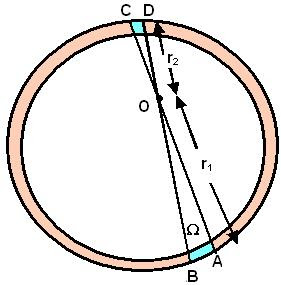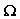## Saturday, September 13, 2008

### Irodov Solution 1.212Solution using Gauss law of gravity :

As in problem 1.211 we can solve this problem using Gauss law of gravity. Clearly if we consider any spherical Gaussian surface inside the spherical shell that passes through A - the point where force is to be evaluated. The total mass contained within the Gaussian sphere is 0. Hence, clearly the gravitational flux at any point within the spherical shell is 0 and consequently so is the force.

An alternate solution using Newton's law of gravitation:Let us try to find the force at some point O within the sphere. Consider the force exerted by an infinitesimally small area (AB) on the shell that subtends a solid anglefrom point O as shown in the figure. The mass spanned by this infinitesimally small area AB will be. Hereis the surface density (mass/unit area) of the spherical shell. On the opposite side of the sphere there will be a corresponding area CD that subtends the same solid angleand its mass will be. The total gravitational force at point O due to AB and CD will be,. Basically what this equation indicates is that if we consider any arbitrary infinitesimally small solid angle from O, the forces due to the two areas subtended by this solid angle on the opposite sides of the spherical shell are equal and opposite. This means that as we integrate over the entire sphere the force is always zero since the opposite sides always cancel!!